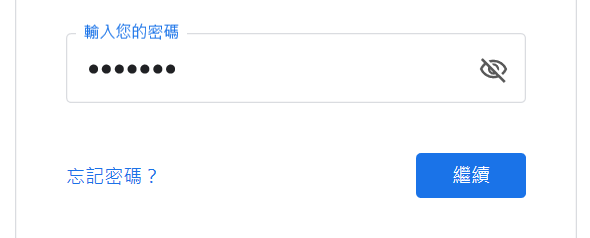#2

## 【python入門教室】(3) 列表和字串也想做加法和乘法?

``````s="HelloHelloHelloHelloHello"
``````

``````s=""
for i in range(5):
s = s + "Hello"
# s 的值變成"HelloHelloHelloHelloHello"
``````

python有很方便的運算子，加法(+)和乘法(*)，

``````s="Hello" * 5
``````

``````>>> "234"+"abc"
'234abc'
>>> "2"+"3"
'23'
>>> "真" * 5 + "高興見到你"
'真真真真真高興見到你'
``````

``````>>> [2,4]+[5,6,9]
[2, 4, 5, 6, 9]
>>>  * 6
[1, 1, 1, 1, 1, 1]
``````

## 範例: 階梯圖形

``````*
**
***
****
*****
******
*******
``````

``````for i in range(1,8):
print('*' * i)
``````

## 範例: 金字塔圖形

``````      *
***
*****
*******
*********
***********
*************
``````

``````for i in range(1,8):
print(' ' * (7-i) + '*' * (2*i-1))
``````

## 範例: 菱形星星

``````      *
***
*****
*******
*********
***********
*************
***********
*********
*******
*****
***
*
``````

``````for i in reversed(range(1,7)):
print(' ' * (6-i) + '*' * (2*i-1))
``````

``````***********
*********
*******
*****
***
*
``````

``````for i in range(1,8):
print(' ' * (7-i) + '*' * (2*i-1))
for i in reversed(range(1,7)):
print(' ' * (7-i) + '*' * (2*i-1))
``````

# 課後練習

## 習題: 密碼不外漏``````def hidePassword(psd):
return #這邊輸入你要回傳的資料
``````

``````輸入: psd = "password"

``````

### 1 則留言

1

``````def hidePassword(psd):
return("*" * len(psd))

n = input("請輸入您的密碼")
``````

``````def hidePassword(psd):
psd = input("請輸入您的密碼")
n = "*" * len(psd)
print(n)

``````

``````def hidePassword(psd):
return "*" * len(psd)

n = input("請輸入您的密碼")
``````

``````def machine(money):
if money==10:
return "奶茶"
if money==20:
return "八寶粥"
``````

`print()`像是伸手去拿販賣機吐出來的東西

(小馬【python入門教室】的課後練習一律採這種形式，在函數不用print, input，只用return，這樣比較單純)

`psd`不是定義在函數的參數中嗎?

python要宣告變數需要透過`=`賦值才算有宣告

``````def machine(x):
return 2*x

machine(x)
``````

`machine`的邏輯只是告訴你當你輸入一個數字，

``````def machine(x):
return 2*x

x = 5
machine(x)
``````

``````def hidePassword(psd):
psd = input("請輸入您的密碼")

``````

Pondudu iT邦新手 5 級 ‧ 2020-04-13 20:36:49 檢舉